# Short Notes: Elasticity of Demand## Concept of Price Elasticity of Demand

• Elasticity means expansion and contraction.
• Elasticity of Demand means expansion and contraction of demand
• Price Elasticity of Demand means expansion and contraction of demand due to change in price.

We know: demand expands (or extends) when price falls, and demand contracts when price rises.

Price elasticity of demand measures the extent to which demand extends when price falls, and the extent to which demand contracts when price rises.

Price elasticity of demand measures the extent to which demand extends when price falls, and the extent to which demand contracts when price rises.

## Measurement of Price Elasticity of Demand

There are three methods of measuring price elasticity of demand:

• Proportionate/Percentage Method
• Total Expenditure or Total Outlay Method
• Geometric Method

Proportionate/Percentage Method

According to this method, price elasticity of demand is measured using the following formula:Example:

At Rs. 46 per unit , the demand for a commodity is 30 units. If the price increases from Rs. 46 to Rs. 50 per unit, the demand decreases from 30 units to 15 units. The price elasticity of demand is:Total Expenditure or Total Outlay Method

Total expenditure on the commodity is measured as the product of price and quantity (Total expenditure = P x Q) when price changes, total expenditure (TE) on the commodity may increase, decrease or remain constant.

Note the following situations:

(i) If, TE remains constant even after change in Px, price elasticity of demand is said to be equal to unity (Ed = 1).

(ii) If TE increases following a fall in Px, and TE decreases following a rise in Px (so that Px and TE move in the opposite direction), price elasticity of demand is said to be greater than unity (Ed = 1).

(iii) If TE increases following an increase in Px and TE decreases following a decrease in Px (so that Px and TE move in the same direction), price elasticity of demand is said to be less than unity (Ed < 1).

 Price Elasticity of Demand (Ed) Px TE Ed = 1(Unitary Elastic) Rises or Falls No ChangeRises Falls Decreases IncreasesRises Falls Increases Decreases

Diagrammatic Presentation: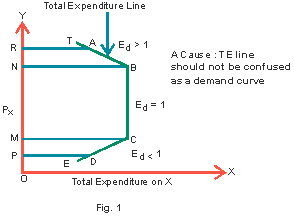Example:

When price of a good falls from Rs. 8 per unit to Rs. 7 per unit, its demand rises from 12 units to 16 units. The elasticity of demand is measured as under.

 Price (Rs) Demand (in units) Total Expenditure (Rs.) 8 12 96 7 16 112

Since, total expenditure increases with fall in price, elasticity of demand is greater than unity. It is a situation of elastic demand.

Geometric Method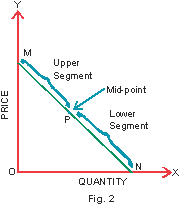This method is used when elasticity of demand is measured corresponding to a specific point on a given demand curve. It is also called ‘Point Method’ of measuring elasticity of demand. This is explained with reference to Fig.2. In this figure, MN is a straight line demand curve. P is a mid-point on the demand curve which divides the demand curve in two equal segments, viz. lower segment (PN) and upper segment (PM). In order to measure elasticity of demand at any point on the demand curve, the lower segment from that point is divided by the upper segment. Thus:Note the following situations:(i): The point of reference happens to be a mid-point on the demand curve, like P in Fig. 3.(ii): If the point of reference happens to be within the upper half of the demand curve, like point A.(iii)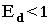: If the point of reference happens to be within the lower half of the demand curve, like point B.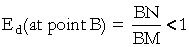(iv)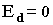: At point N, where the demand curve touches the X-axis,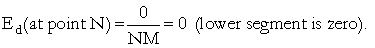(v)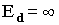: At point M, where the demand curve touches the Y-axis,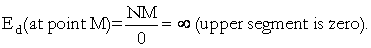## Degrees of Price Elasticity of Demand

The five cases of elasticity of demand depending upon their degree are as under:1. Perfectly Elastic Demand: A perfectly elastic demand refers to a situation when demand is infinite at the prevailing price. It is a situation where the slightest rise in price causes the quantity demanded of the commodity to fall to zero. In Fig. 4, DD is the perfectly elastic demand curve which is parallel to OX-axis. In this case, elasticity of demand is infinite or Ed = ∞.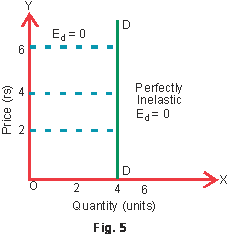2. Perfectly Inelastic Demand: A perfectly inelastic demand refers to a situation when change in price has no effect on quantity demanded i.e., demand remains unchanged. It is a situation when even substantial change in price leaves the demand unaffected. In Fig.5, DD is the perfectly inelastic demand curve which is parallel to OY-axis. Elasticity of demand is zero or Ed = 0.3. Unitary Elastic Demand: When change in quantity demanded in response to change in price of the commodity is such that total expenditure on the commodity remains constant implying a situation of unitary elastic demand. Here, elasticity of demand is unity or Ed = 1. Fig. 6 shows the situation of unitary elastic demand.

In this case, TE before the change in price(OBTP) = TE after a fall in price (OCRP1)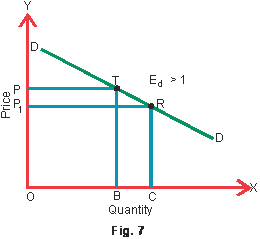4. Greater than Unitary Elastic: Demand is more than unitary elastic when change in quantity demanded in response to change in price of the commodity is such that total expenditure on the commodity increases and decreases when price increases. Fig. 7 shows the situation of unitary elastic demand. Here, elasticity of demand is greater than unity or Ed > 1.

In this case, TE before the change in price(OBTP) 1)5. Less than Unitary Elastic: Demand is less than unitary elastic when change in quantity demanded in response to change in price of the commodity is such that the total expenditure on the commodity decreases when price falls, and increases when price rises. Fig. 8. shows the situation of less than unitary elastic demand. Here, elasticity of demand is less than unitary or Ed < 1.

In this case, TE before the change in price(OBTP) > TE after a fall in price (OCRP1)

If two demand curves intersect each other, then at the point of intersection, flatter the curve more elastic it is.

Refer to Fig. 9. In the figure, demand curves D1D2 and D2D2 are intersecting each other at point.At the point of intersection i.e., at point A, OP = initial price and OQ = initial quantity for both the demand curves D1D2 and D2D2.

At point A,is same for both the demand curves D1D2 and D2D2 which is equal to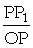.

Consequently, elasticity of demand can be compared by comparing the ratio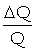.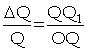in case of demand curvein case of demand curve D2D2.

We can observe that OQ2 > OQ1           [∴OQ on D1D1 = OQ on D2D2]

Implying that elasticity of demand is greater at point A for demand curve D2D2(flatter curve) than for demand curve D1D1.

At the common point of intersection, elasticity of demand is higher for the flatter curve.

## Factors Determining the Price Elasticity of Demand

1. Nature of Commodity: Ordinarily, demand for necessities like salt, matchboxes, Kerosene oil, etc. is less than unitary elastic or inelastic. It is so because change in their price has little effect on their demand. On the other hand, demand for luxuries, is greater than unity i.e. elastic. Price, elasticity of demand is neither very elastic nor very inelastic in case of comforts i.e. milk, cooler, fans, etc.

2. Availability of Substitutes: The greater the substitutes available for the product, the greater will be its elasticity of demand. Commodities that do not have any substitutes like liquor, cigarettes, etc. have inelastic demand.

3. Goods with Different Uses: The greater the number of uses to which a commodity can be put, the greater is its elasticity of demand.

4. Postponement of the Use: Goods whose demand can be postponed to a future period have elastic demand. On the other hand, goods whose demand cannot be postponed have inelastic demand.

5. Habit of the Consumer: Goods to which a person becomes habitual will have inelastic demand like cigarette, tobacco, coffee, etc. It is so because a person cannot do without them.

6. Price Level: Elasticity of demand also depends upon the level of price of the concerned commodity. Elasticity of demand will be high at higher level of the price of the commodity and low at the lower level of the price.

7. Time Period: Demand is inelastic in short period but elastic in long period. It is so because in the long-run, a consumer can change his habits more conveniently than in the short period.

## Some Useful Illustrations

1. When price of the good increases from Rs. 10 to Rs. 15 per unit, total expenditure on the commodity increases from Rs. 1,000 to Rs. 1,500. Find elasticity of demand.

Ans. P = Rs 10, P1 = Rs 15

TE = Rs 1,000, TE1 = Rs 1500

The elasticity of demand is less than one or commodity has inelastic demand because in response to increase in price, total expenditure has also risen even when quantity demanded decreases.

2. There is 50% fall in price of the commodity. But quantity demanded remains to be 150 units. Find elasticity of demand.

Ans. Here, elasticity of demand is zero because in response to decrease in price of the commodity, the quantity demanded remains the same, i.e., 150 units.

3. A certain quantity of the commodity, is purchased when its price is Rs. 10 per unit. Quantity demanded increases by 50% in response to a fall in price by Rs. 2 per unit. Find elasticity of demand.

Ans. Percentage change in quantity demanded = 504. At a certain price of the commodity quantity purchased is 150 units. When price falls by 25%, quantity purchased increases by 75 units. Find elasticity of demand.

Ans. Percentage change in price = ? 255. Price elasticity of demand is found to be (?)2. Price falls from Rs. 10 per unit to Rs. 8 per unit. Find the percentage change in quantity demanded.

Ans. Given, Ed = (?) 2, P = Rs 10, P1 = Rs 8Percentage change in quantity demanded = (?) 2 _ ? 20

= 40

6. For a commodity, ΔP/P = -0.2, and elasticity of demand = ? 0.3. Find percentage change in quantity demanded.Percentage change in quantity demanded = 0.3 _ 20 = 6

7. For a commodity, ΔP/P = -0.2, and elasticity of demand is ? 0.5. Find quantity demanded after a fall in price when initially it was 60 units.

Ans. Given,Initial quantity demanded (Q) = 60 unitsNew quantity, Q1 = 60 + 6 = 66 units.

8. A commodity shows Ed = (-)2, Quantity demanded reduces from 300 units to 150 units. In response to increase in price. Find the increased price when initially it was Rs. 20 per unit.

Ans.9. Given Ed = -0.2 and percentage increase in quantity demanded = 20%, find the percentage change in expenditure.

Ans.10. If Ed = -0.4 and0.5, find new expenditure on the commodity if initial expenditure is Rs. 1,500.

Ans.11. When price of a good rises from Rs. 5 per unit to Rs. 6 per unit, its demand falls from 20 units to 10 units. Compare expenditures on the good to determine whether demand is elastic or inelastic.

Ans.

 Price (Rs) Demand (in units) Total Expenditure (Rs.) 5 20 100 6 10 60

Here, total expenditure decreases with rise in price, hence elasticity of demand is more than unity. It is a situation of elastic demand.

12. Price elasticity of demand of a good is (?)1. At a given price the consumer buys 60 units of the good. How many units will the consumer buy if the price falls by 10 per cent?

Ans.The consumer will purchase 66 units of the good when price falls by 10 percent.

13. Price elasticity of demand of a good is (?) 4. If the price increases from Rs. 10 per unit to Rs. 12 per unit, what is the percentage change in demand?

Ans.Hence, the percentage change in demand = (?) 80 or demand decreases by 80 per cent.

14. At a given market price of a good a consumer buys 100 units. When price increases by 40 per cent he buys 80 units. Calculate price elasticity of demand.

Ans.15. A consumer buys a certain quantity of a good at a price of Rs. 10 per unit. When price falls to Rs. 8 per unit, she buys 40 per cent more quantity. Calculate price elasticity of demand by percentage method.

Ans.Thus, price elasticity of demand = 2